StatLect

# EM algorithm

The Expectation-Maximization (EM) algorithm is a recursive algorithm that can be used to search for the maximum likelihood estimators of model parameters when the model includes some unobservable variables (also called latent variables).## Latent-variable model

In a latent-variable model, there are two vectors:

• the observed data;

• the vector of unobserved variables.

We denote their joint probability bywhereis a vector of parameters to be estimated.

## Model specification

The joint distribution ofandis usually obtained by separately specifying the conditional distribution of the observed variablesand the marginal distribution of the latent variablesAs a consequence, the joint distribution isIn the context of latent-variable models, the joint distribution is often called complete likelihood.

## Derived quantities

Given the model specification above, ifis a discrete random vector, we can derive the marginal distribution ofaswhere the sum is over the setof all the values thatcan possibly take (its support).

We can also derive the conditional distribution ofasIfis continuous the sums above are replaced by integrals.

## Maximum likelihood problem

The maximum likelihood estimator (MLE) of the parameteris the vectorthat solves the maximization problemThis problem does not usually have an analytical solution. As a consequence, we need to solve it with an iterative procedure that starts from an initial guessof the solution and produces a sequencethat hopefully converges to the solution.

We say hopefully because we are often unable to derive theoretical guarantees about the numerical convergence of likelihood maximization algorithms, especially for latent-variable models.

The EM algorithm is one of the iterative procedures that can be used to search for a solution when we are dealing with a latent-variable model specified as above.

## The algorithm

Starting from an initial guess, the-th iteration of the EM algorithm consists of the following steps:

1. use the parameter valuefound in the previous iteration to compute the conditional probabilitiesfor each;

2. use the conditional probabilities derived in step 1 to compute the expected value of the complete log-likelihood:3. solve the maximization problem4. if the parameter update is smaller than a pre-specified threshold, that is, ifstop the algorithm, else return to step 1.

Steps 1 and 2 are collectively called the Expectation step, while step 3 is called the Maximization step. Hence the name of the algorithm (Expectation-Maximization).

Step 4 is a stopping criterion: we stop the algorithm when there are no significant changes in the parameter.

## Theoretical guarantees

In general, the algorithm is not guaranteed to converge to a global maximum of the likelihood.

However, it has the important property that the likelihood is guaranteed to increase at each iteration:for every.

Proof

The conditional probability formulaimplies thatIf we multiply both sides by, we obtainThen, we can sum over the support of:Since the log-likelihooddoes not depend onandwe have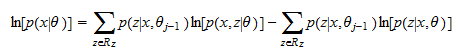Moreover,Thus,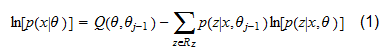Setting, we obtainSubtracting (2) from (1), we get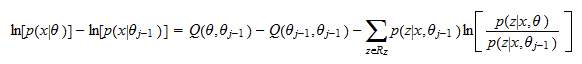By Jensen's inequality, we have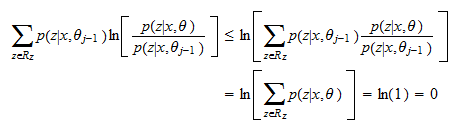Therefore,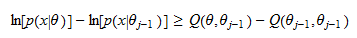which, for, becomes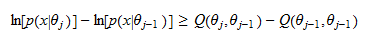ButbecauseThereforeor## Advantages of the EM algorithm

The EM algorithm is particularly advantageous when the maximization problem in the Maximization stephas a closed-form solution.

This happens, for example, when the latent-variable model is a mixture of multivariate normal distributions.

## Caveats

As we said, there is no guarantee that the EM algorithm converges to a global maximum of the likelihood.

If we suspect that the likelihood may have multiple local minima, we should use the multiple starts approach. In other words, we should run the EM algorithm several times with different starting values.

## Example: estimation of Gaussian mixtures

The EM algorithm is often used to estimate the parameters of a mixture of normal distributions (also called Gaussian mixture). See this lecture for details.